Скачать презентацию A Weighted Average of Sparse Several Representations is

cd2d52437cec724a04576da72a76570d.ppt

• Количество слайдов: 24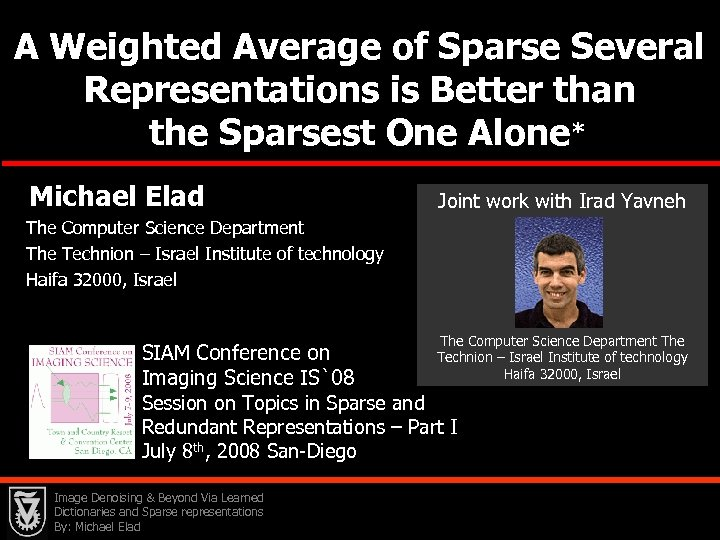A Weighted Average of Sparse Several Representations is Better than the Sparsest One Alone* Michael Elad Joint work with Irad Yavneh The Computer Science Department The Technion – Israel Institute of technology Haifa 32000, Israel SIAM Conference on Imaging Science IS`08 Session on Topics in Sparse and Redundant Representations – Part I July 8 th, 2008 San-Diego Image Denoising & Beyond Via Learned Dictionaries and Sparse representations By: Michael Elad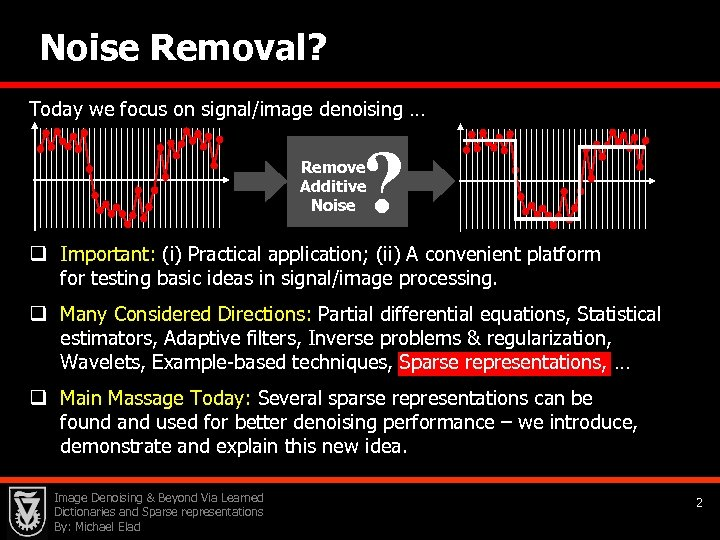Noise Removal? Today we focus on signal/image denoising … ? Remove Additive Noise q Important: (i) Practical application; (ii) A convenient platform for testing basic ideas in signal/image processing. q Many Considered Directions: Partial differential equations, Statistical estimators, Adaptive filters, Inverse problems & regularization, Wavelets, Example-based techniques, Sparse representations, … q Main Massage Today: Several sparse representations can be found and used for better denoising performance – we introduce, demonstrate and explain this new idea. Image Denoising & Beyond Via Learned Dictionaries and Sparse representations By: Michael Elad 2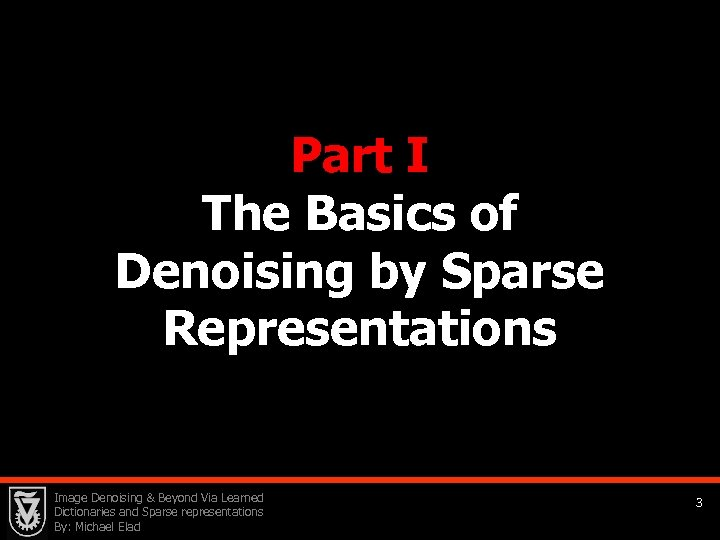Part I The Basics of Denoising by Sparse Representations Image Denoising & Beyond Via Learned Dictionaries and Sparse representations By: Michael Elad 3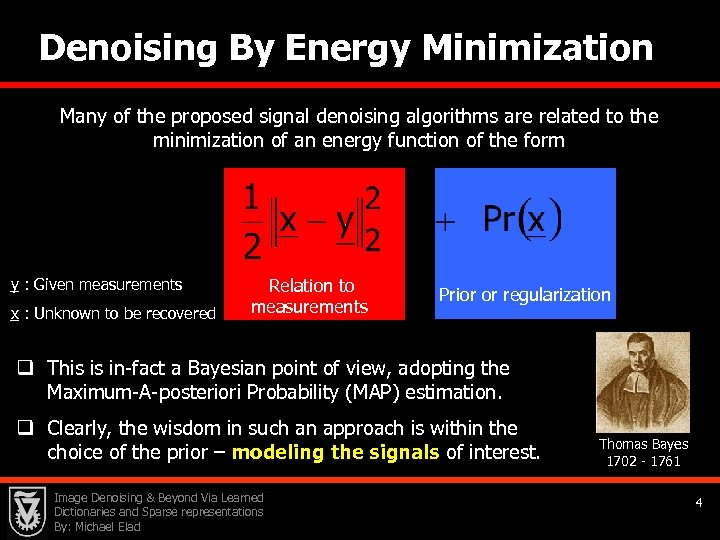Denoising By Energy Minimization Many of the proposed signal denoising algorithms are related to the minimization of an energy function of the form y : Given measurements x : Unknown to be recovered Relation to measurements Prior or regularization q This is in-fact a Bayesian point of view, adopting the Maximum-A-posteriori Probability (MAP) estimation. q Clearly, the wisdom in such an approach is within the choice of the prior – modeling the signals of interest. Image Denoising & Beyond Via Learned Dictionaries and Sparse representations By: Michael Elad Thomas Bayes 1702 - 1761 4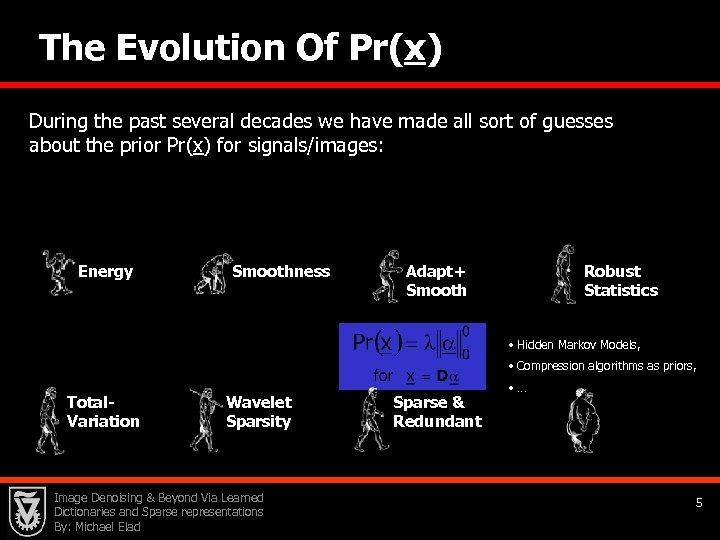The Evolution Of Pr(x) During the past several decades we have made all sort of guesses about the prior Pr(x) for signals/images: Energy Smoothness Adapt+ Smooth Robust Statistics • Hidden Markov Models, • Compression algorithms as priors, Total. Variation Wavelet Sparsity Image Denoising & Beyond Via Learned Dictionaries and Sparse representations By: Michael Elad Sparse & Redundant • … 5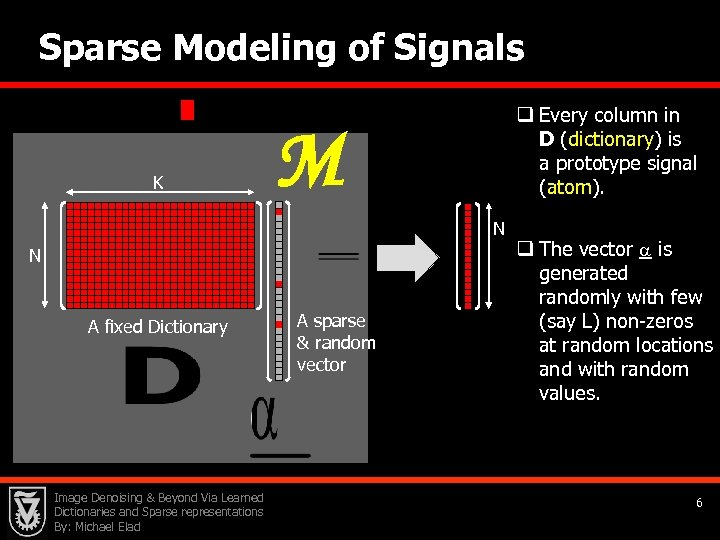Sparse Modeling of Signals K q Every column in D (dictionary) is a prototype signal (atom). M N N A fixed Dictionary Image Denoising & Beyond Via Learned Dictionaries and Sparse representations By: Michael Elad A sparse & random vector q The vector is generated randomly with few (say L) non-zeros at random locations and with random values. 6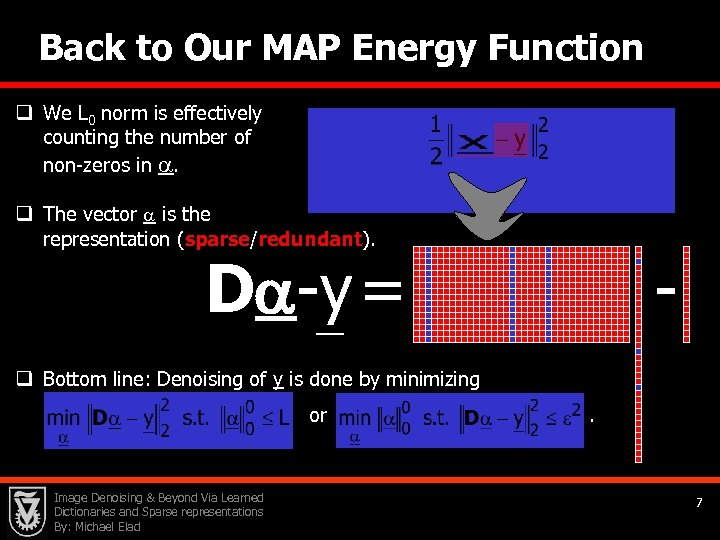Back to Our MAP Energy Function q We L 0 norm is effectively counting the number of non-zeros in . q The vector is the representation (sparse/redundant). D -y = - q Bottom line: Denoising of y is done by minimizing or Image Denoising & Beyond Via Learned Dictionaries and Sparse representations By: Michael Elad . 7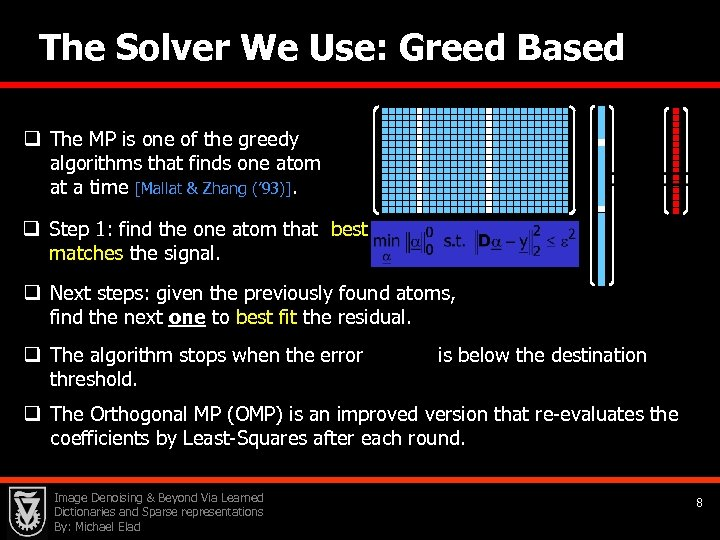The Solver We Use: Greed Based q The MP is one of the greedy algorithms that finds one atom at a time [Mallat & Zhang (’ 93)]. q Step 1: find the one atom that best matches the signal. q Next steps: given the previously found atoms, find the next one to best fit the residual. q The algorithm stops when the error threshold. is below the destination q The Orthogonal MP (OMP) is an improved version that re-evaluates the coefficients by Least-Squares after each round. Image Denoising & Beyond Via Learned Dictionaries and Sparse representations By: Michael Elad 8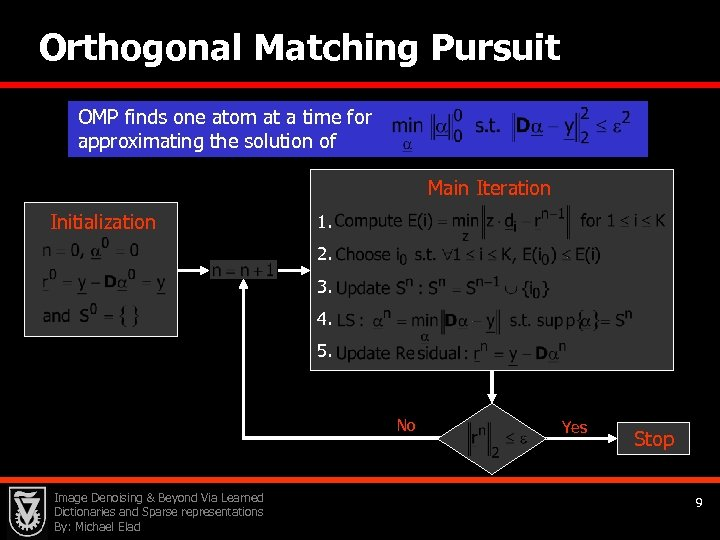Orthogonal Matching Pursuit OMP finds one atom at a time for approximating the solution of Main Iteration Initialization 1. 2. 3. 4. 5. No Image Denoising & Beyond Via Learned Dictionaries and Sparse representations By: Michael Elad Yes Stop 9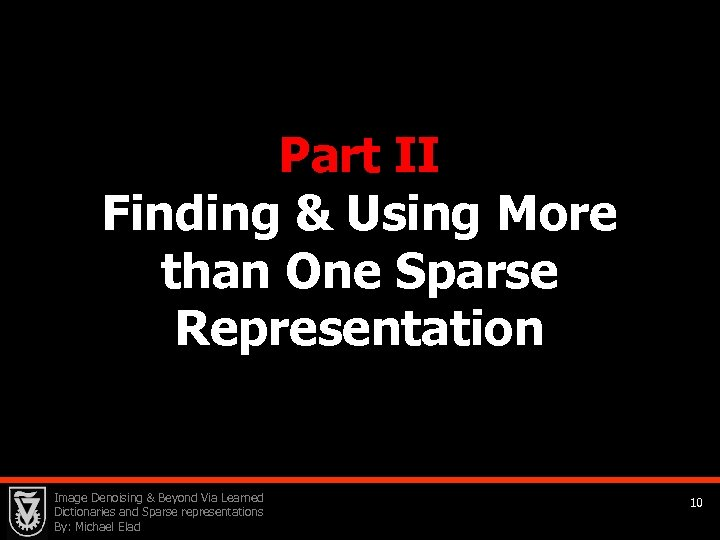Part II Finding & Using More than One Sparse Representation Image Denoising & Beyond Via Learned Dictionaries and Sparse representations By: Michael Elad 10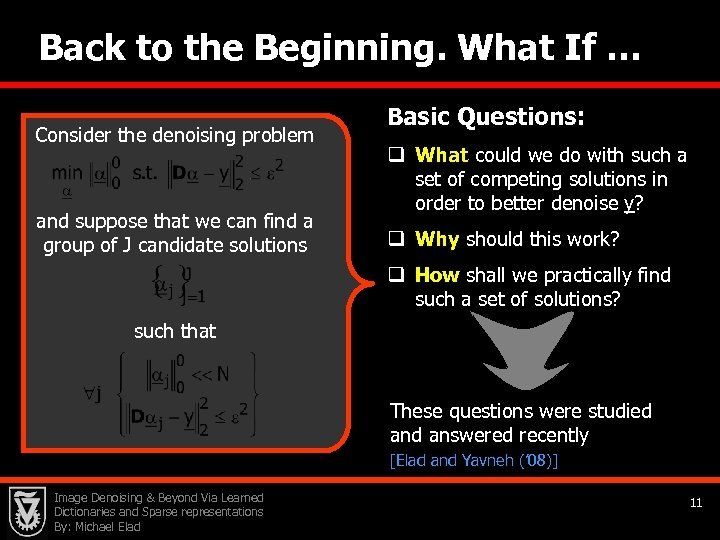Back to the Beginning. What If … Consider the denoising problem and suppose that we can find a group of J candidate solutions Basic Questions: q What could we do with such a set of competing solutions in order to better denoise y? q Why should this work? q How shall we practically find such a set of solutions? such that These questions were studied answered recently [Elad and Yavneh (’ 08)] Image Denoising & Beyond Via Learned Dictionaries and Sparse representations By: Michael Elad 11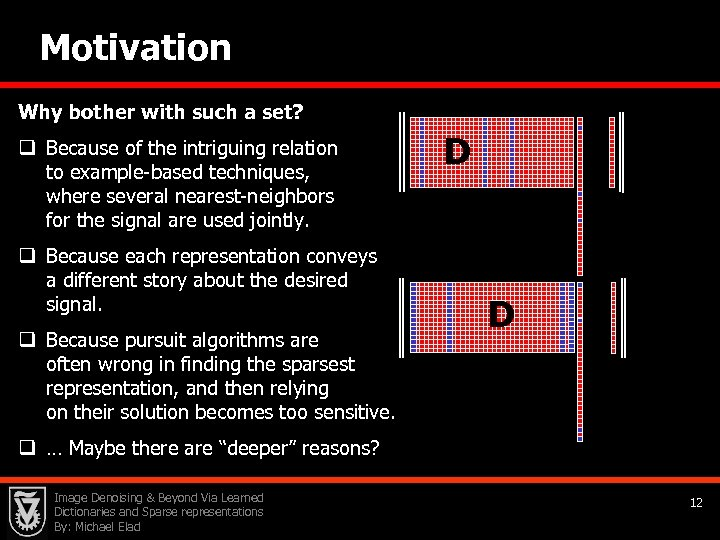Motivation Why bother with such a set? q Because of the intriguing relation to example-based techniques, where several nearest-neighbors for the signal are used jointly. q Because each representation conveys a different story about the desired signal. q Because pursuit algorithms are often wrong in finding the sparsest representation, and then relying on their solution becomes too sensitive. D D q … Maybe there are “deeper” reasons? Image Denoising & Beyond Via Learned Dictionaries and Sparse representations By: Michael Elad 12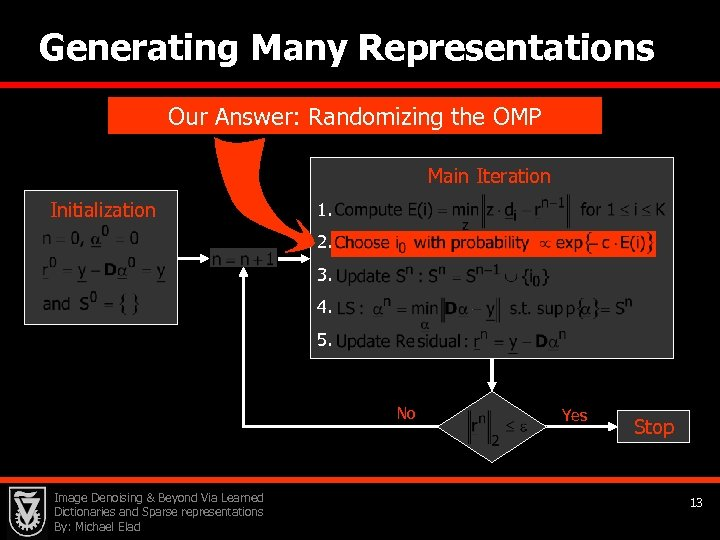Generating Many Representations Our Answer: Randomizing the OMP Main Iteration Initialization 1. 2. 3. 4. 5. No Image Denoising & Beyond Via Learned Dictionaries and Sparse representations By: Michael Elad Yes Stop 13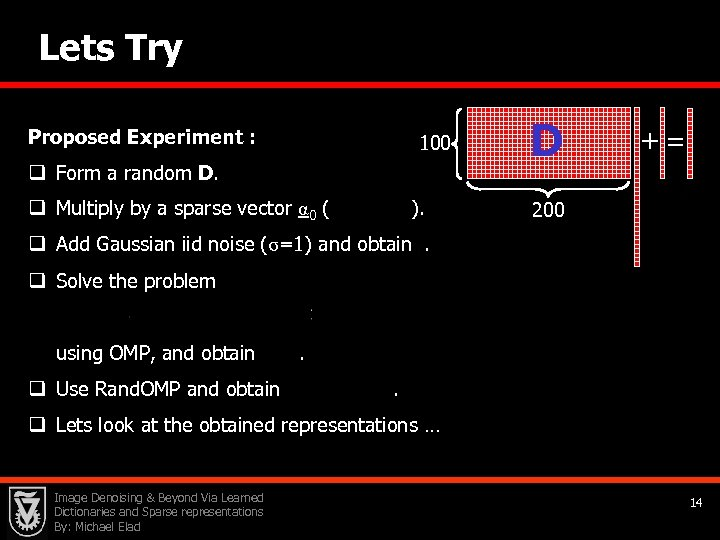Lets Try Proposed Experiment : 100 q Form a random D. q Multiply by a sparse vector α 0 ( ). D += 200 q Add Gaussian iid noise (σ=1) and obtain. q Solve the problem using OMP, and obtain q Use Rand. OMP and obtain . . q Lets look at the obtained representations … Image Denoising & Beyond Via Learned Dictionaries and Sparse representations By: Michael Elad 14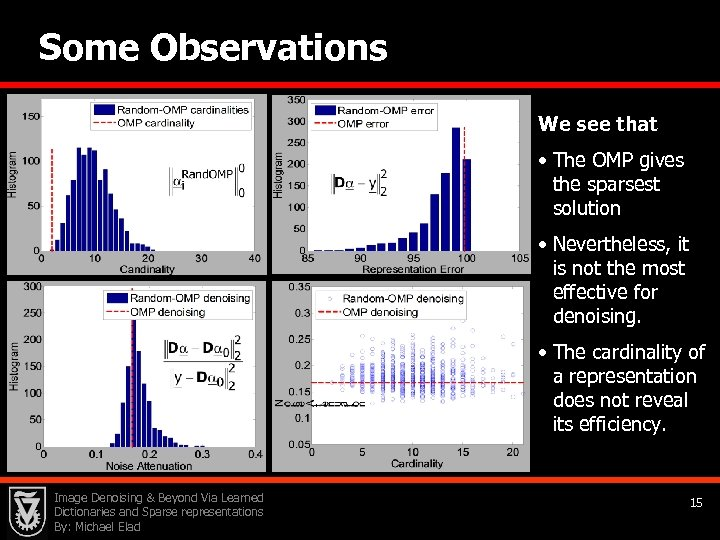Some Observations We see that • The OMP gives the sparsest solution • Nevertheless, it is not the most effective for denoising. • The cardinality of a representation does not reveal its efficiency. Image Denoising & Beyond Via Learned Dictionaries and Sparse representations By: Michael Elad 15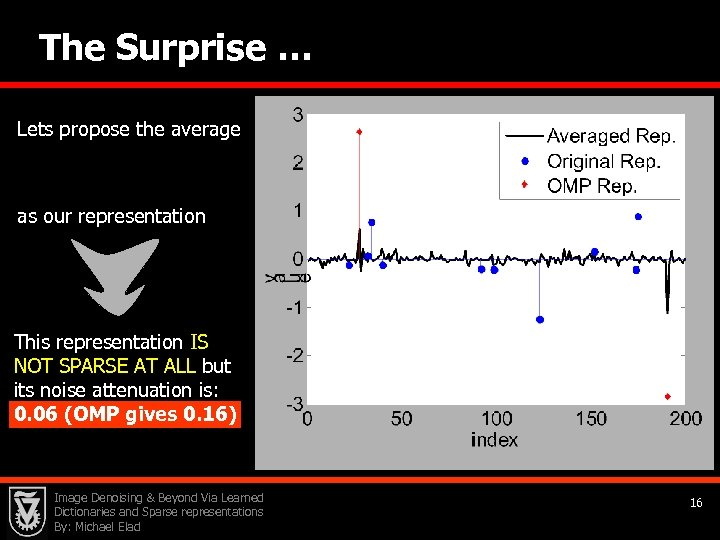The Surprise … Lets propose the average as our representation This representation IS NOT SPARSE AT ALL but its noise attenuation is: 0. 06 (OMP gives 0. 16) Image Denoising & Beyond Via Learned Dictionaries and Sparse representations By: Michael Elad 16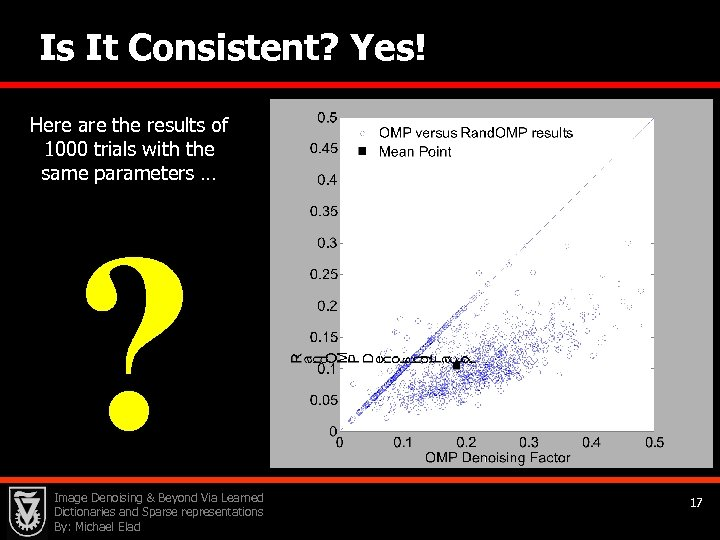Is It Consistent? Yes! Here are the results of 1000 trials with the same parameters … ? Image Denoising & Beyond Via Learned Dictionaries and Sparse representations By: Michael Elad 17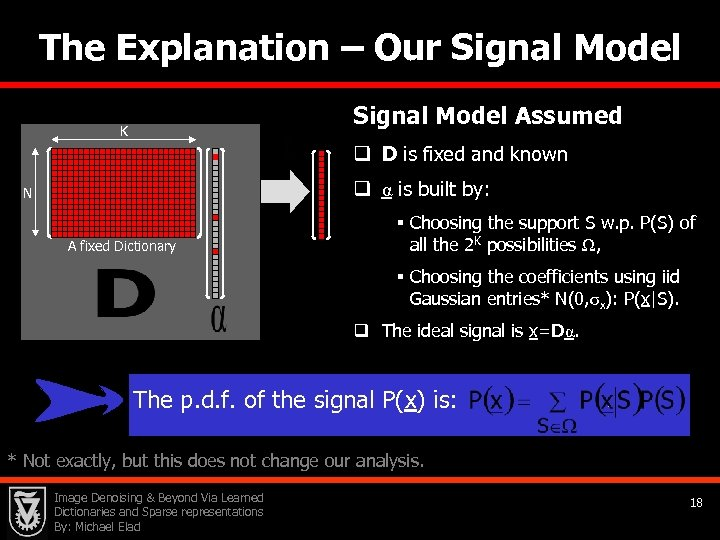The Explanation – Our Signal Model Assumed K q D is fixed and known q α is built by: N A fixed Dictionary § Choosing the support S w. p. P(S) of all the 2 K possibilities Ω, § Choosing the coefficients using iid Gaussian entries* N(0, σx): P(x|S). q The ideal signal is x=Dα. The p. d. f. of the signal P(x) is: * Not exactly, but this does not change our analysis. Image Denoising & Beyond Via Learned Dictionaries and Sparse representations By: Michael Elad 18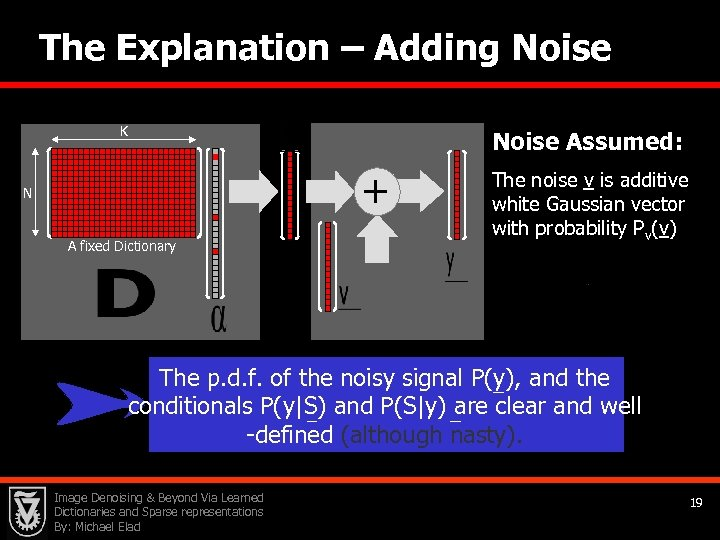The Explanation – Adding Noise K Noise Assumed: + N A fixed Dictionary The noise v is additive white Gaussian vector with probability Pv(v) The p. d. f. of the noisy signal P(y), and the conditionals P(y|S) and P(S|y) are clear and well -defined (although nasty). Image Denoising & Beyond Via Learned Dictionaries and Sparse representations By: Michael Elad 19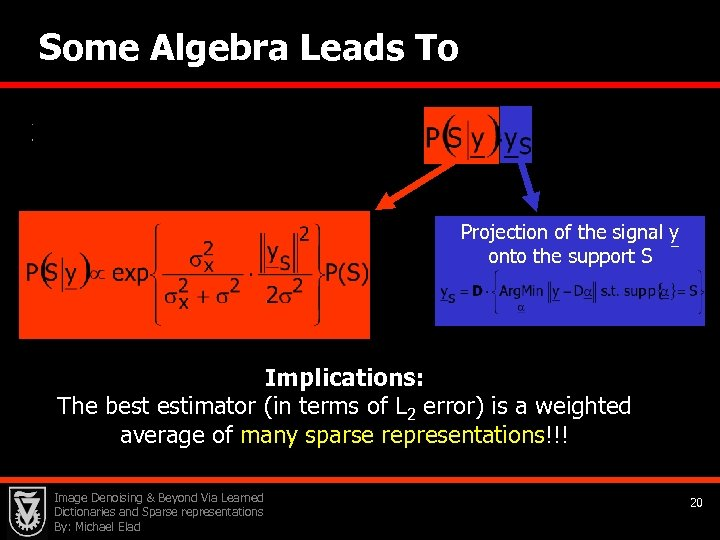Some Algebra Leads To Projection of the signal y onto the support S Implications: The best estimator (in terms of L 2 error) is a weighted average of many sparse representations!!! Image Denoising & Beyond Via Learned Dictionaries and Sparse representations By: Michael Elad 20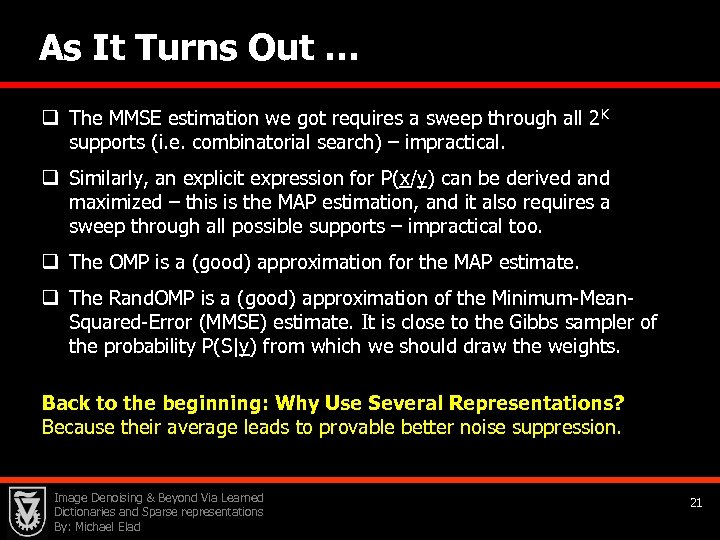As It Turns Out … q The MMSE estimation we got requires a sweep through all 2 K supports (i. e. combinatorial search) – impractical. q Similarly, an explicit expression for P(x/y) can be derived and maximized – this is the MAP estimation, and it also requires a sweep through all possible supports – impractical too. q The OMP is a (good) approximation for the MAP estimate. q The Rand. OMP is a (good) approximation of the Minimum-Mean. Squared-Error (MMSE) estimate. It is close to the Gibbs sampler of the probability P(S|y) from which we should draw the weights. Back to the beginning: Why Use Several Representations? Because their average leads to provable better noise suppression. Image Denoising & Beyond Via Learned Dictionaries and Sparse representations By: Michael Elad 21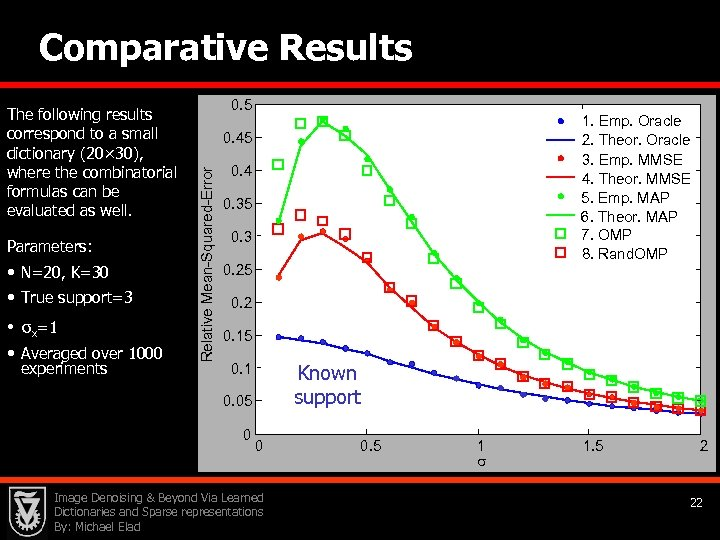Comparative Results Parameters: • N=20, K=30 • True support=3 • σx=1 • Averaged over 1000 experiments 0. 5 1. Emp. Oracle 2. Theor. Oracle 3. Emp. MMSE 4. Theor. MMSE 5. Emp. MAP 6. Theor. MAP 7. OMP 8. Rand. OMP 0. 45 Relative Mean-Squared-Error The following results correspond to a small dictionary (20× 30), where the combinatorial formulas can be evaluated as well. 0. 4 0. 35 0. 3 0. 25 0. 2 0. 15 Known support 0. 1 0. 05 0 0 Image Denoising & Beyond Via Learned Dictionaries and Sparse representations By: Michael Elad 0. 5 1 s 1. 5 2 22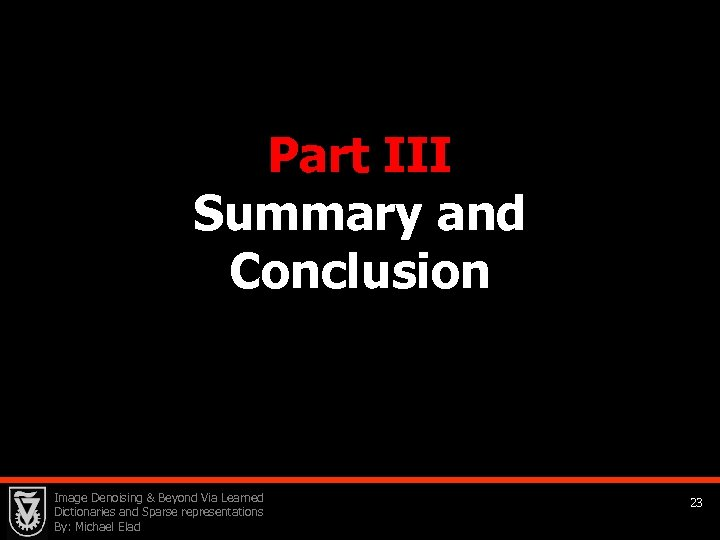Part III Summary and Conclusion Image Denoising & Beyond Via Learned Dictionaries and Sparse representations By: Michael Elad 23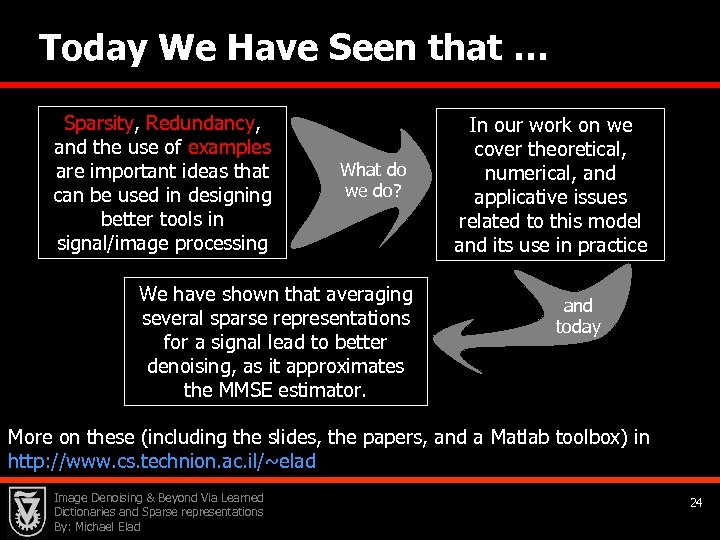Today We Have Seen that … Sparsity, Redundancy, and the use of examples are important ideas that can be used in designing better tools in signal/image processing What do we do? We have shown that averaging several sparse representations for a signal lead to better denoising, as it approximates the MMSE estimator. In our work on we cover theoretical, numerical, and applicative issues related to this model and its use in practice and today More on these (including the slides, the papers, and a Matlab toolbox) in http: //www. cs. technion. ac. il/~elad Image Denoising & Beyond Via Learned Dictionaries and Sparse representations By: Michael Elad 24